# What Are Watts and Why Do They Matter?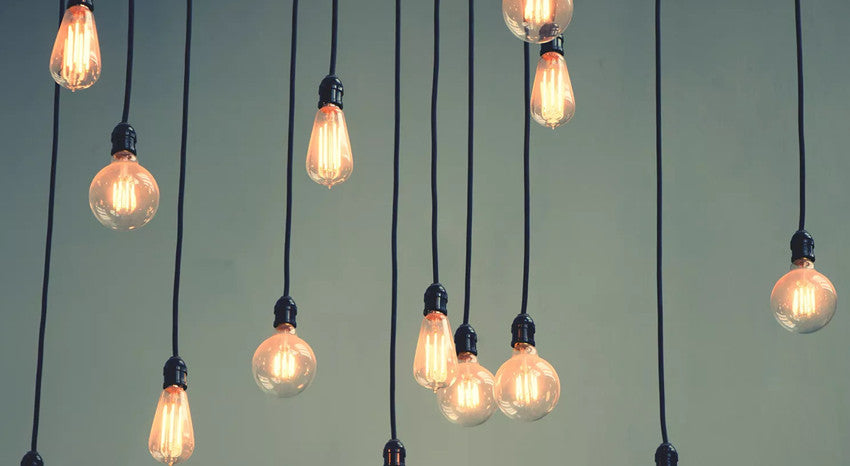How many watts does an RV refrigerator use? Which one consumes more power– a conventional halogen light bulb or an LED bulb? How many watts of solar energy do I need to run a fan all night on my boat?

Watts matter to us all day and all night, even when we sleep. But what is the abstract thing called Watt, and why do we need to know about them? In this article, we are going to show you why understanding watts is more important than you think.

## What is Watt In Simple Terms?

Electrical power is measured in watts. A watt is a unit of power. When we use the term Watt, we are putting a number on the rate of energy transfer. That's meaning, one watt is a single unit of power, and power is the rate at which energy is created or consumed by an object.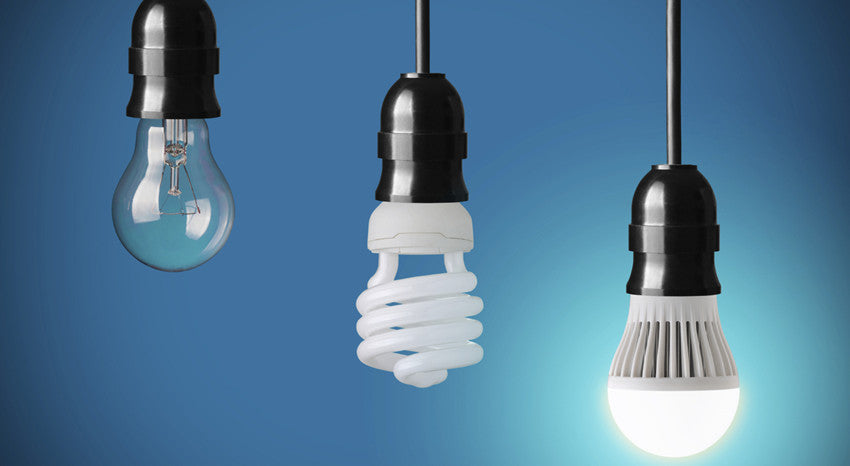If we think of watts as measuring electrical flow, then we can think of any device or appliance as requiring either a large electrical flow or a small electrical flow. For example, if you have a 100-watt light bulb and a 60-watt light bulb, you can think of the 100-watt bulb as requiring a larger flow of energy to work. If you want to run a 750-watt microwave oven, you are going to need a larger flow of energy – 750 watts to be exact.

In the same way, if you have solar panels on the roof of your RV, the solar energy flowing into the RV is measured in watts.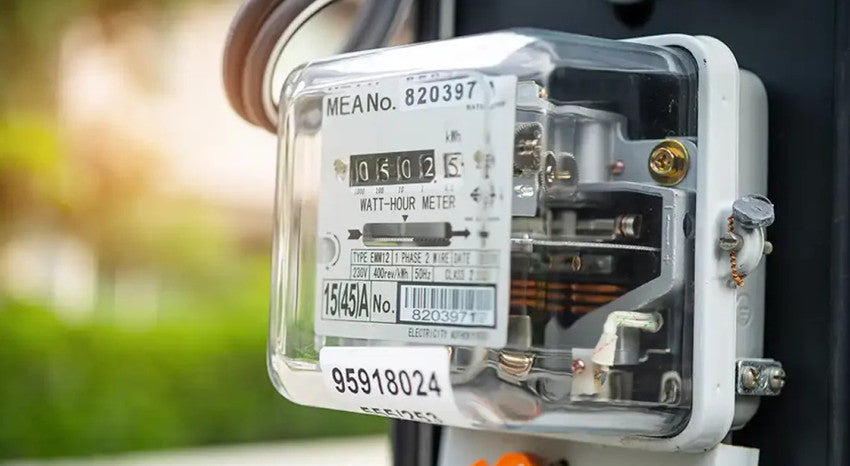## Common Watt Multiples and What They Mean

Watts are measured in multiples of 1,000. You have probably heard of these multiples before, but let’s look at what they mean in terms of power. The smallest measurement typically used is a milliwatt or 1/1000th of a watt. This measurement is commonly used in small circuits such as computers or cell phones.

Here we only see milliwatts of electricity flowing through these tiny wires.

A kilowatt is a unit of measure for 1,000 watts of electrical power. Abbreviated as a kilowatt (kW), the kilowatt is a globally recognized standard for measuring electricity energy. You may notice that your home’s energy usage or electricity consumption is rated in kilowatts on your electric bill.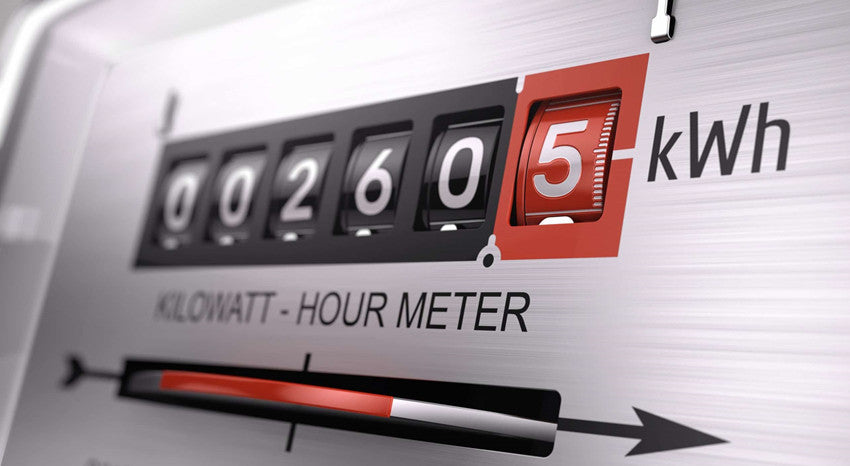One megawatt (MW) is globally recognized to be equal to 1,000 kilowatts of power. Depending on the size of the generator, the power rating of the generator can be megawatts, kilowatts, or watts.

Any device in your home that runs on electricity will have its usage or consumption rated in watts or kilowatts. In the same way, a light bulb might be rated at 60 watts, while a microwave is rated at 750 watts.

Interestingly, some appliances have two different ratings. For example, our household refrigerator is an appliance that has a start (or surge) rating and a running rating. This means that the wattage will be different when the refrigerator first starts up versus when it has been running for a while.

It might have a 1,200 watts startup rating and an 800 watts running capacity rated. Thus, when the refrigerator starts (when the compressor kicks on), it needs 1,200 watts at that moment. But while it is running normally, the required wattage drops to 800 watts.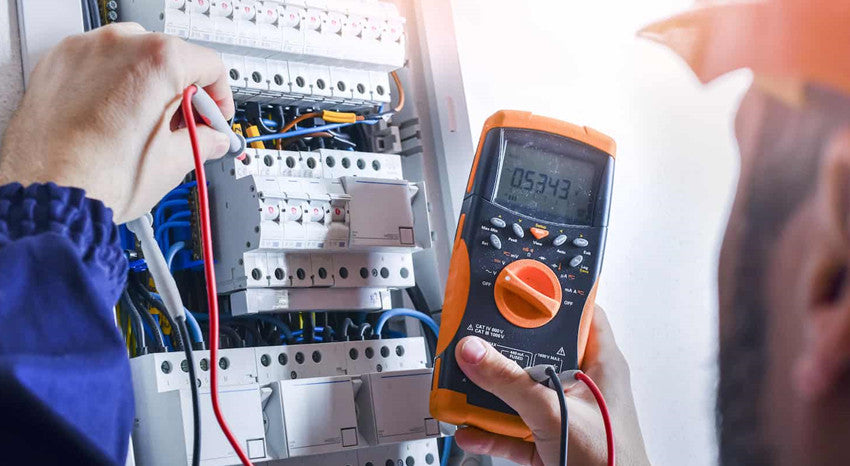## How Do You Measure Watts?

There is a simple formula we can learn to help us measure watts in any situation. To be able to calculate the number of watts provided by a power supply, you will need to know the number of amps and volts in that power source. You can use a multimeter to measure amps and volts. Once you have this information, the calculation of the DC circuit is simple:

Watts(W) = Amps(A) x Volts(V). So, let's assume the current is 6 amps, and the voltage is 110 volts, then 6 x 110 = 660 watts.

You will also sometimes see this equation written as Power = Amps x Volts ( P = I x V). This is a more formal or technical version of the formula, which you might see in a textbook. However, as long as you can remember watts = amps x volts, you will be good to go for DC circuits.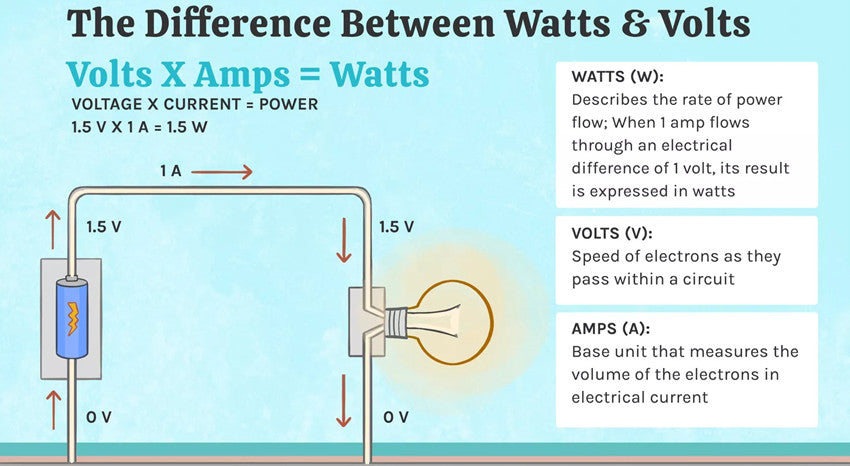## High Wattage and Low Wattage

High wattage means more power is consumed.  Because of this, we spent most of the time trying to build and use appliances that consume less power and have lower wattage. However, higher wattage is good when you particularly need to convert electricity into heat. Because the higher the wattage the hotter the heater.

However, it is important to know that high wattage can mean high heat. When you are powering high-wattage equipment, more heat is produced, which needs to be accounted for within the context of your particular application. High-wattage electronic devices can also cause a circuit overload and blow a fuse or trip a circuit breaker.

Conversely, low-wattage applications require less power to charge or operate. Such as the low-wattage of the cell phone charger, the smartphone or tablets, and the LED light bulb.

Also, keep in mind that it doesn’t matter whether we are talking about a 12-volt or a 220-volt application. A watt is a watt, and it is not the same property as voltage. A device doesn't consume less power just because it is a 12 volts device.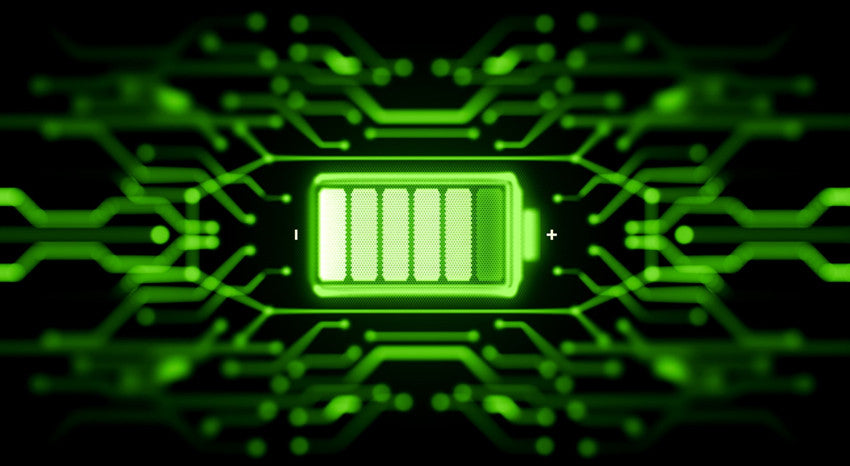## Power Supply Capacity

The job of a power source is to provide electric energy to the connected appliance. So, it would make sense that the more power an appliance requires, the higher the wattage you will need from the power supply. (Higher wattage = more power).

There is a rule of thumb to keep in mind when considering the capacity of a power supply. It is better to have more power than you need because you shouldn’t be running your power supply at 100% capacity.

For example, if you want to run a 100 watts device, you would not use a 100-watt power source. Why? As we mentioned earlier, you don’t want to run your power source at full capacity. Ideally, you would want your power supply to have a wattage higher than 100 in this situation.

If you have a 250-watt power supply and you use it to run a 100-watt appliance, that power supply will only put out the necessary 100 watts the appliance needs. Essentially, the power supply won't "Overpower" the appliance.## Why Do Watts Matter in RVs and Boats?

When you are traveling on a boat or in an RV, watts matter because you need to be able to calculate your daily electricity requirements. For marine applications, it is helpful to know how much power your motor needs to be based on the size of your boat. In RVs, you will need to understand what appliances you can power and whether you can power more than one appliance at a time.

For example, RVs are often powered by using generators. If your generator puts out 4,000 watts of power, you might be able to run your RV’s microwave oven, air conditioner, and several small appliances, although maybe not all at once.

## How many watts of infographic

If you are considering installing a solar system on your boat or RV, you will need to be able to calculate how much power (how many watts) you are using over a 24-hour period. This will help you understand how many solar panels you will need (based on their wattage) to be able to sustain your daily usage. We will share how to calculate this at the end.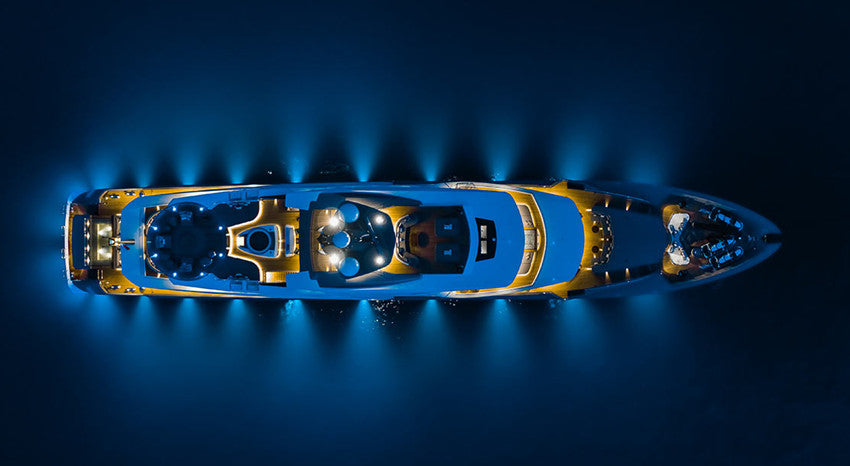## Where Will You See Watts In RVs & Boats?

RVs and boats have many applications that require power, from lights to device chargers to fans, microwave ovens, and refrigerators. It is important to understand how many watts you will consume in a day, and how you are going to recharge your batteries to store more energy for the next day.

## Power Conservation

Using lower-wattage equipment where possible is key, especially when you are not connected to shore power (i.e. living off-grid). Let’s take lights as an example to illustrate the variations in power consumption. Remember, watts = power.

Let's assume an incandescent bulb that illuminates at a level of 450 lumens will use 40 watts. On the other hand, an LED light illuminated at the same level will only use 4-5 watts. If you are living off-grid, and mostly rely primarily on solar energy, you are probably concerned about your electricity consumption. In this case, which bulb would you prefer to have in your rig or on your boat? The LED bulb uses only a few watts, of course.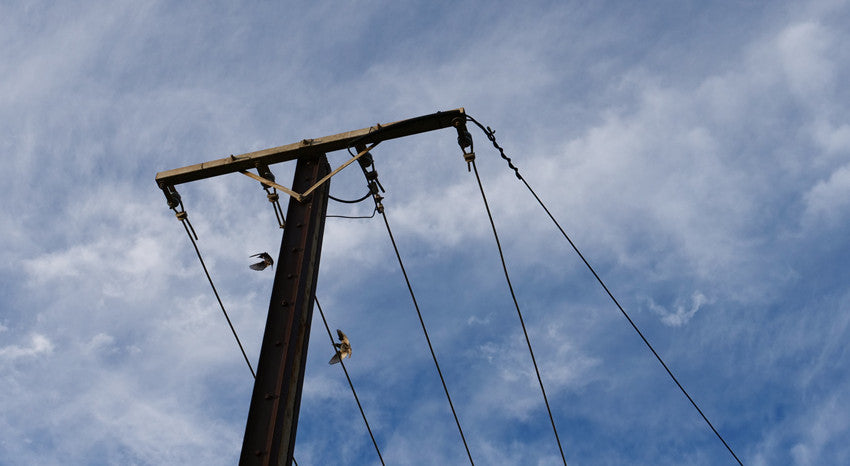## Inverters

When you are considering appliances that use more power, you may need an inverter to run them. Inverters convert DC current into AC current to run certain appliances and equipment.

DC current is the type of electrical current you receive from a battery (think powering an RV). AC current is the type of electricity you receive from a power station (think powering a residential home).

An inverter needs to have sufficient capacity to power what you want it to power. So, in order to know what inverter you will require, you have to know the wattage (starting and running) of the equipment you want to power.

Let’s go back to our refrigerator example at the beginning of this article. If the refrigerator starts at 1,200 watts of capacity and runs at 800 watts of capacity, a 750-watt inverter would be insufficient to run this appliance. Instead, you would want an inverter with a larger capacity, like 1,500 watts.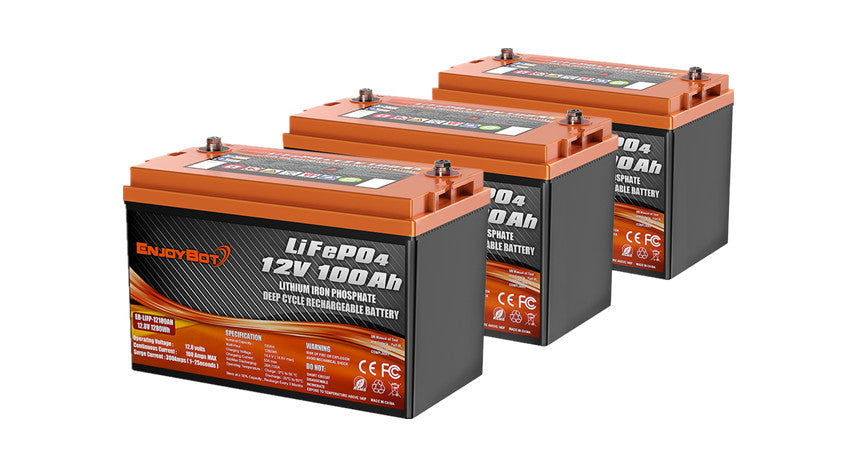Another thing that needs to be considered with inverters is that most inverters are rated in VA or volt-ampere.  In an AC circuit, there is something called a power factor that causes the power to appear higher than the load to the generator or source.  This is a complicated topic but to account for this it's typically good to add an extra 20% margin to your loads to get an accurate power consumed.

## Generators and Solar Panels

Now let’s suppose you are in the market for a portable generator to give your off-grid adventures a power boost. If you want to run an air conditioner in the summer heat, your generator is going to need to be able to provide sufficient power to start and run the air conditioner unit. This is true of everything you want to electric energy.

And finally, if you are looking at investing in solar panels to harness the energy of the sun, you need to be able to calculate how many watts of solar energy you require to power your off-grid life. How many watts of solar energy you need will be determined by how many watts of solar you use.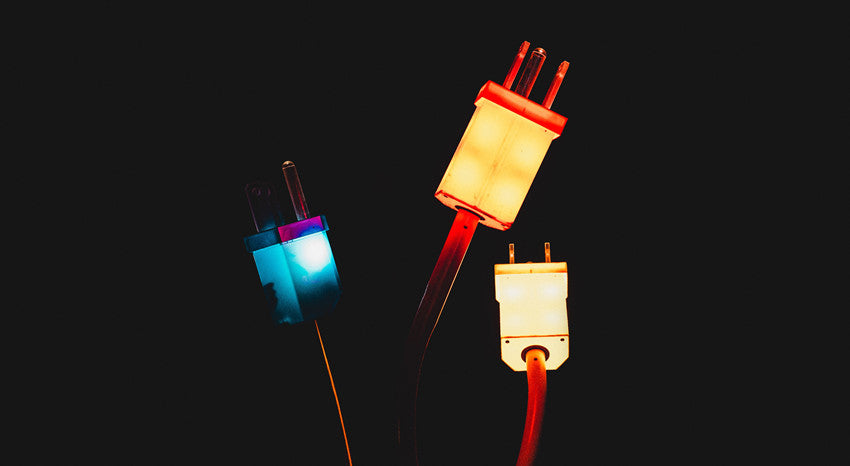## Distinction Between Watts and Watt-Hours

The difference between watts and watt-hours is simple. The electrical power is measured in watts. Watts measures the rate of power at a specific moment in time.

Watt-hour measures the rate of power over a specific period (one hour).

Simply put, one watt-hour equals one watt of power flow in an hour. So, a 5-watt LED light bulb lighting on for one hour has consumed 5-watt hours of energy.

## How Many Watts of Solar Do You Need?

To illustrate the significance of watts and watt-hours, let’s calculate how many watts of solar panels you will need to power your boat or RV off-grid.

First, make a list of all the electrical equipment you will want to run. Then, write down the wattage of each electric unit and how long you intend to run each one. Next, total the wattage of all devices by adding them all together, then calculate the sum of the run times for all the devices.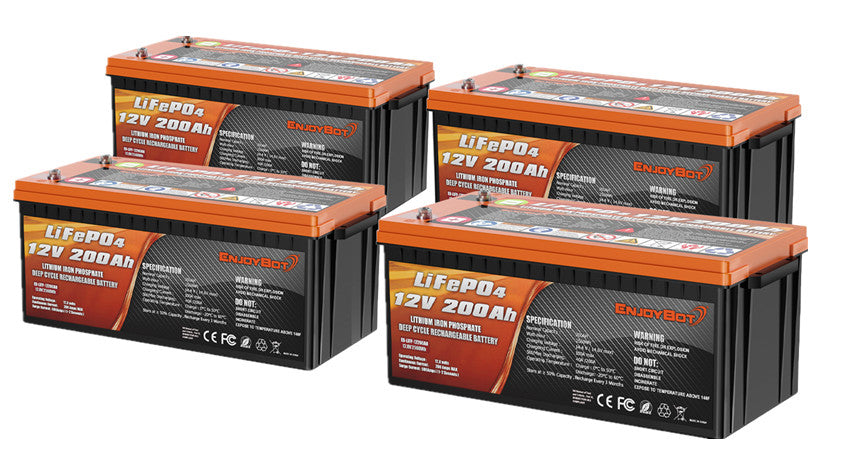Finally, multiply these two totals together (total wattage x total estimated running time). The result tells you how many watt-hours are required to power all of your electrical devices with solar panels.

Let's assume you are planning to run all of your equipment for 24 hours. If you have a solar panel rated at 250-watt, your panel can produce 6,000 watt-hours (or 6 kilowatt-hours) of power during that time (250 x 24 = 6,000). Meaning, if the total watt-hour requirement you calculated for all of your devices over a 24-hour period is 6,000 watts, then you will need more than one solar panel to accommodate your power needs.

## Conclusion

How many watts of solar power do you need? How much solar power do you need? Watt does matter!

Watts are very significant in any application where electricity is required, whether that is in a home, business, large facility, boat, or RV. Simply stated, watts matter – and so does understanding them!

You have successfully subscribed!
This email has been registered